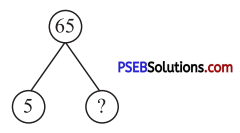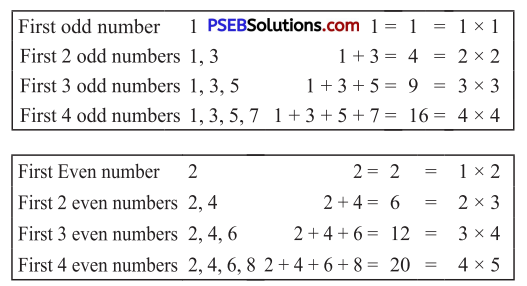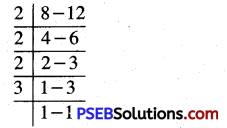# PSEB 5th Class Maths MCQ Chapter 3 HCF and LCM

Punjab State Board PSEB 5th Class Maths Book Solutions Chapter 3 HCF and LCM MCQ Questions and Answers.

## PSEB 5th Class Maths Chapter 3 HCF and LCM MCQ Questions

Tick (✓) the right answer :

Question 1.
Which number is the smallest even Prime number ? ………
(a) 0
(b) 1
(c) 2
(d) 4
(c) 2

Question 2.
Which number is neither Prime nor Composite ?
(a) 1
(b) 2
(c) 3
(d) 4
(a) 1Question 3.
Which numbers are Prime numbers between 70 and 80 ?
(a) 71, 72, 73
(b) 71, 75, 79
(c) 71, 80
(d) 71, 73, 79.
(d) 71, 73, 79.

Question 4.
HCF of 75 and 90 = ……
(a) 5
(b) 10
(c) 15
(d) 20.
(c) 15

Question 5.
LCM of 12, 18 and 24 = …….
(a) 72
(b) 36
(c) 48
(d) 24
(a) 72

Question 6.
If HCF of any two numbers is 8 then out of following which can not be LCM of that numbers ?
(a) 48
(b) 60
(c) 24
(d) 56
(b) 60

Question 7.
What is length of the largest tape which can measure the lengths of 24 m and 30 m ?
(a) 4 m
(b) 5 m
(c) 6 m
(d) 7 m.
(c) 6 m

Question 8.
What is the smallest number which is divisible by 8 and 12 ?
(a) 16
(b) 48
(c) 72
(d) 24
(d) 24

Question 9.
LCM of 26 and 39 = ……
(a) 13
(b) 78
(c) 39
(d) 26

Question 10.(a) 5
(b) 65
(c) 12
(d) 13.
(d) 13.

Question 11.
Which number is composite number in the following?
(a) 43
(b) 23
(c) 21
(d) 37.
(c) 21Question 12.
Out of following, which number is multiple of 19?
(a) 171
(b) 172
(c) 173
(d) 174.
(a) 171

Question 13.
HCF of 15, 45 and 105 = ………
(a) 15
(b) 5
(c) 30
(d) 45.
(a) 15

Question 14.
What is the HCF of two prime numbers ?
(a) 1
(b) 2
(c) 3
(d) 4
(a) 1

Question 15.
Three bells ring with the time gap of 10 min, 15 min and 20 min respectively in a school. If all bells are rung together at 9:00 am then after how long the bells would ring together again ?
(a) 11:00 o’clock
(b) 08:00 o’clock
(c) 10:00 o’clock
(d) 12:00 o’clock
(c) 10:00 o’clockQuestion 16.
Read the above pattern and find the sum of first 6 Odd numbers.
(a) 30
(b) 12
(c) 25
(d) 36.
(d) 36.

Question 17.
Read the above pattern and find the sum of first 10 Odd numbers.
(a) 20
(b) 50
(c) 100
(d) 40.
(c) 100

Question 18.
Read the above pattern and find the sum of first 8 Even numbers.
(a) 16
(b) 24
(c) 72
(d) 64.
(c) 72

Question 19.
Read the above pattern and find sum of first 9 even numbers.
(a) 19
(b) 18
(c) 45
(d) 90.
(d) 90.Question 20.
On a given road, the poles are erected at a distance of 24 m each and piles of stones are lying at a distance of 30 m each. If the 1st pile of stones is lying adjacent to the first pole, at what distance will the pole and the pile of stones be together again ?
(a) 100 m
(b) 110 m
(c) 150 m
(d) 120 m.
(d) 120 m.

Question 21.
What is the length of the largest inchitape which can measure the lengths of 24 m and 30 m completely:
(a) 4 m
(b) 5 m
(c) 6 m
(d) 7 m.